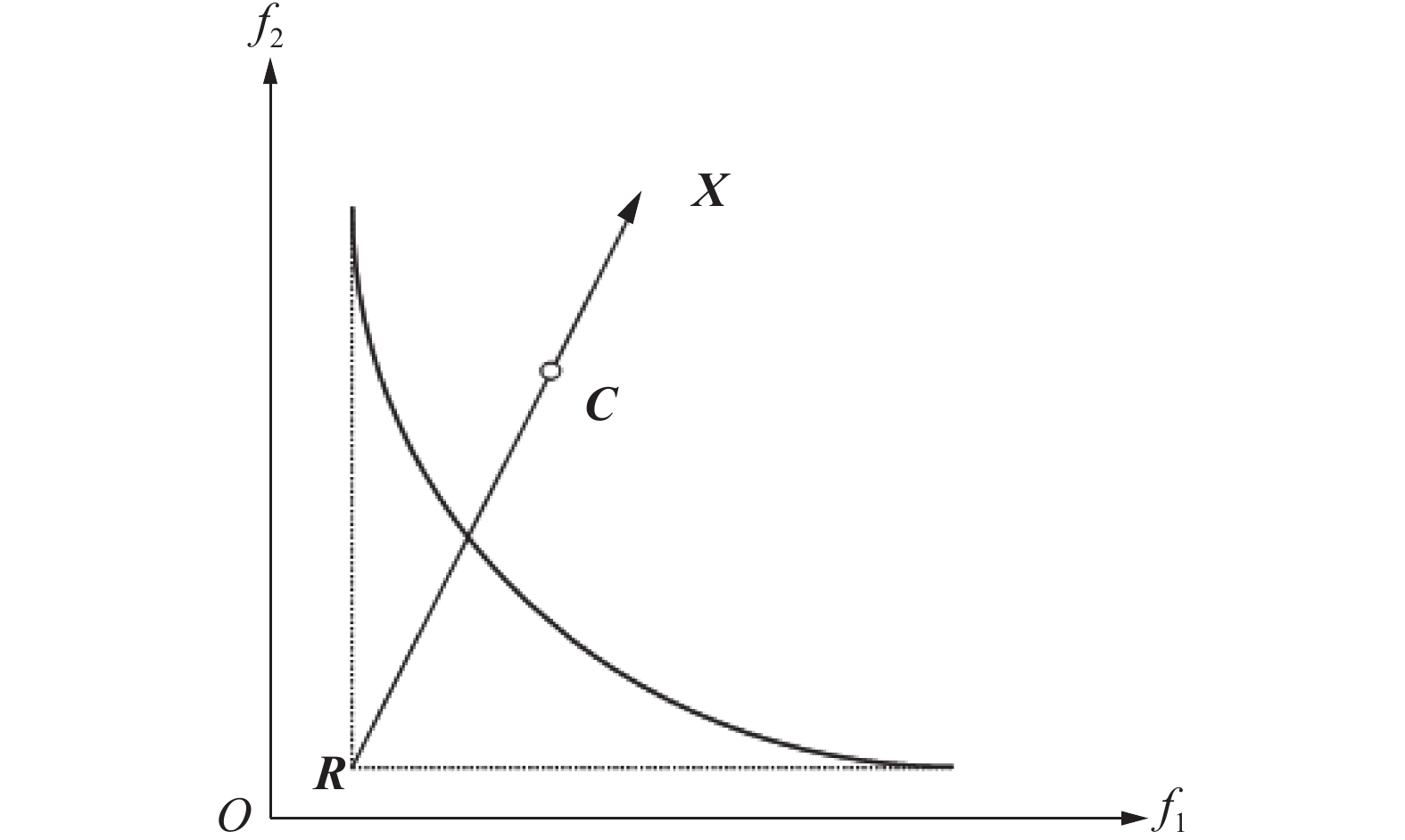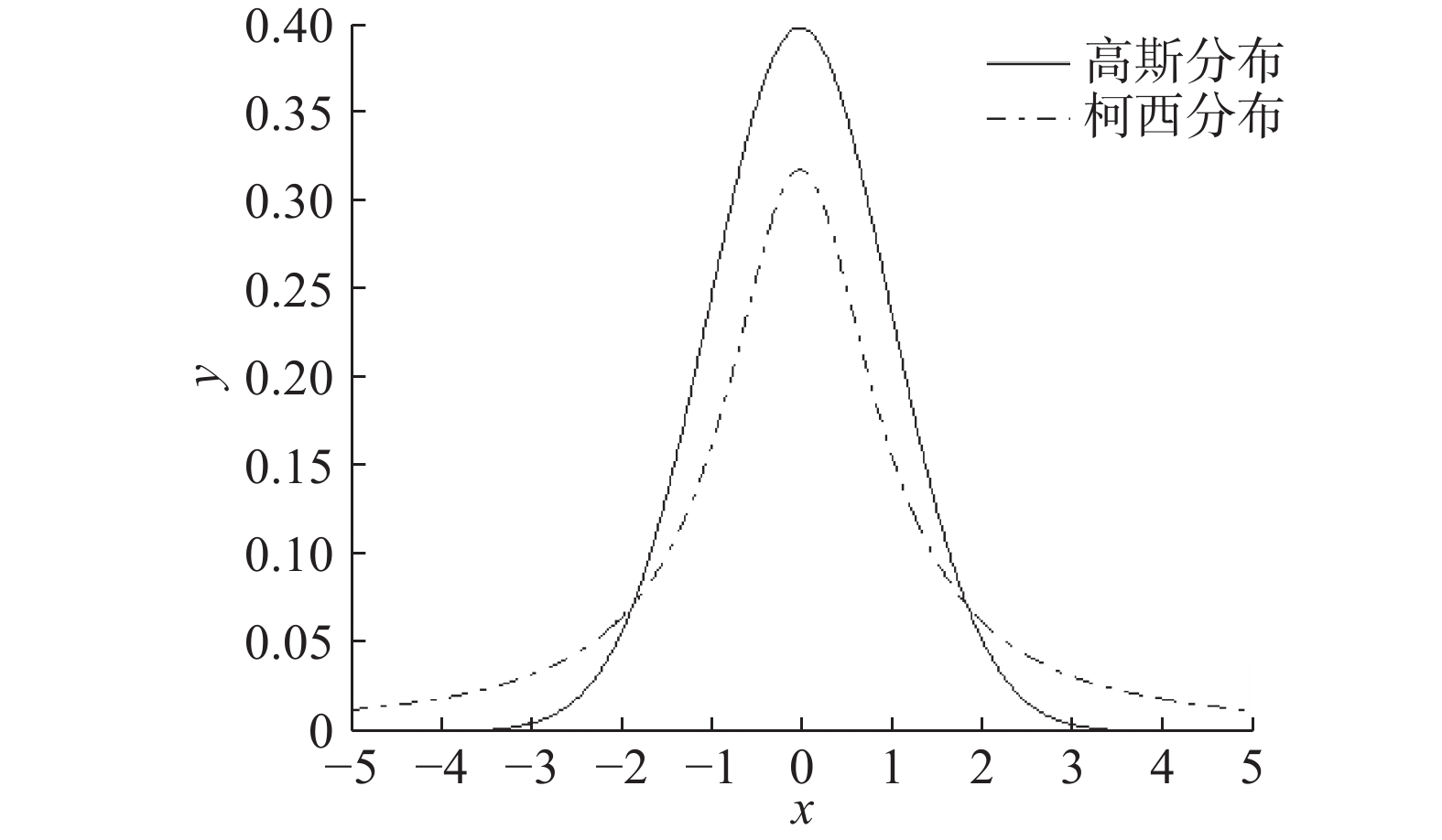﻿ 基于目标空间分解和连续变异的多目标粒子群算法
«上一篇文章快速检索 高级检索

 智能系统学报2019, Vol. 14Issue (3): 464-470  DOI: 10.11992/tis.2017110150

### 引用本文QIAN Xiaoyu, GE Hongwei, CAI Ming. Decomposition and continuous mutation-based multi-objective particle swarm optimization[J]. CAAI Transactions on Intelligent Systems, 2019, 14(3): 464-470. DOI: 10.11992/tis.201711015.### 文章历史

1. 江南大学 轻工过程先进控制教育部重点实验室，江苏 无锡 214122;
2. 江南大学 物联网工程学院，江苏 无锡 214122;
3. 江南大学 信息化建设与管理中心，江苏 无锡 214122

Decomposition and continuous mutation-based multi-objective particle swarm optimization
QIAN Xiaoyu 1,2, GE Hongwei 1,2, CAI Ming 3
1. Ministry of Education Key Laboratory of Advanced Process Control for Light Industry, Jiangnan University, Wuxi 214122, China;
2. School of Internet of Things Engineering, Jiangnan University, Wuxi 214122, China;
3. Information Construction and Management Center, Jiangnan University, Wuxi 214122, China
Abstract: In light of the poor convergence problems and the diversity of current multi-objective optimization algorithms, in this paper, we propose an objective-space decomposition and continuous mutation-based multi-objective particle-swarm-optimization algorithm. Its innovations are as follows : we use a space decomposition method to distribute the particle swarm into a predefined sub-region. During this process, we apply a new adaptive value formula to select and filter the particles in each sub-region and incorporate a fitness formula into the dominance factor. In the global search process, we apply differential, Gaussian, and Cauchy mutations to continuously mutate the position of the global guide particle. We compare the performance of this algorithm with those of current multi-objective optimization algorithms, and the results show that the proposed algorithm improves the convergence and diversity of the particles.
Key words: multi-objective optimization    particle swarm optimization algorithm    decomposition    sub-region    mutation    differential    Gaussian mutation    Cauchy mutation

1 多目标优化问题 1.1 基本概念
 $\left\{ {\begin{array}{*{20}{l}} {\min y = F(x) = ({f_1}(x),{f_2}(x),\cdots,{f_m}(x))} \\ {{\rm{s.t}}.{g_i}(x) \leqslant 0,\;i = 0,1,2,\cdots,q} \\ {{h_j}(x) = 0,\;j = 1,2,\cdots,p} \end{array}} \right.$ (1)

 $\left\{ {\begin{array}{*{20}{c}} {\forall i \in \{ 1,2,\cdots,m\},\,\,\, {f_i}(d) \leqslant {f_i}(e)} \\ {\exists i \in \{ 1,2,\cdots,m\},\,\,\, {f_i}(d) < {f_i}(e)} \end{array}} \right.$ (2)

 ${\rm PS} = \{ x \in \varOmega |\neg \exists z \in \varOmega ;z \succ x\}$ (3)

 ${\rm{PF}} = \{ F(x)|x \in {\rm{PS}}\}$ (4)
1.2 粒子群优化

 $V_k^{t + 1} = WK_k^t + {C_1}{R_1}(P - X_k^t) + {C_2}{R_2}(G - X_k^t)$ (5)
 $X_k^{t + 1} = V_k^{t + 1} + X_k^t$ (6)

2 MOPSO/DC算法 2.1 目标空间分解

 $\begin{split} u = & \displaystyle\sum\limits_{{k_1} = 0}^h {\displaystyle\sum\limits_{{k_2} = 0}^{h - {k_1}}\cdots}\\ & \displaystyle\sum\limits_{{k_i} = 0}^{h - {k_1} - \cdots - {k_{i - 1}}} \cdots\displaystyle\sum\limits_{{k_{m - 3}} = 0}^{h - {k_1} - \cdots - {k_{m - 4}}} {{{\left[\displaystyle\sum\limits_{{k_{m - 2}} = 1}^{h - {k_1} - \cdots - {k_{m - 3}}} {(h + 2 - \displaystyle\sum\limits_{l = 1}^{m - 2} {{k_l}} ) + {k_{m - 1}} + 1} \right]} } } \end{split}$

2.2 粒子的分类与更新Download: 图 1 粒子的方向向量 Fig. 1 Direction vector of the particle

1)有的子区域粒子数大于子区域的容量Vol时，通过适应值进行取舍，适应值公式为

 $f = aT + {\rm{CD}}$ (7)

2)当子区域粒子数少于该子区域容量Vol时，由于情况1)中已经对劣质的粒子进行删除，为了尽可能保证整体的优越性，所以重新初始化该子区域所缺数目的粒子作为该区域的粒子，增加获得较优粒子的可能性，增加粒子多样性。然后计算新粒子的目标函数值。

2.3 连续变异操作

 $\left\{ \begin{gathered} {X_{{\rm{diff}}}} = X + 0.5({X_1} - {X_2}) \hfill \\ {X_{{\rm{Cauchy}}}} = \frac{{c{{(t)}^2}}}{{\text{π} ({X^2} + c{{(t)}^2})}} \hfill \\ {X_{{\rm{Gauss}}}} = \frac{1}{{g(t)\sqrt {2\text{π} } }}\exp (\frac{{ - {X^2}}}{2}) \hfill \\ \end{gathered} \right.$ (8)Download: 图 2 标准高斯和分布柯西分布对比 Fig. 2 Comparison of standard Gaussian and Cauchy distributions

2.4 MOSPO/DC步骤

1)初始化2N个粒子的位置，这些粒子的位置集合记为POP，初始化N个速度，这些速度集合记为V，设目标函数的个数为m，当前迭代次数为t，初始化最大迭代次数gmax、每个子区域容量 ${\rm{Vol}} \in [1,3]$ 、邻域个数T以及子区域数目M

2)进行目标空间分解操作。

3)计算粒子群POP目标函数值，选出每个目标函数的最小值作为参考点R用于计算每个粒子的方向向量，方向向量的计算参考图1

4)进行粒子的分类与更新操作，接着将当前所有子区域粒子的位置信息存入集合EPOP中，最后更新参考点R，并重新计算每个粒子的方向向量，清空POP备用。

5)gbest的选择：产生[0，1]之间的随机数r1,当r1>0.8时，随机从EPOP集合中选择一个粒子作为当前粒子的gbest；否则，在该粒子所在区域的邻域内操作。首先计算该粒子邻域中每个子区域的中心向量和该子区域中粒子方向向量的余弦值，然后选出余弦值最大的子区域中的粒子作为当前粒子的gbest。过多地使用变异会让算法变得更加随机，降低了算法的效率，所以产生[0,1]之间的随机数r2,当r2<0.6时，对选出的引导粒子位置进行连续变异操作，确定最终的引导粒子gbest。否则，不对gbest进行变异操作。

6)pbest的选择：产生[0,1]之间的随机数r3，当r3>0.8时，随机从EPOP中选择一个粒子；否则，从该粒子的所在区域的邻域中随机选择一个粒子。让当前粒子和这个随机选出的粒子进行比较，选出支配权优先的作为当前粒子的pbest

7)通过上述选出的gbestpbest，以及粒子速度更新式(5)和位置更新式(6)产生下一代新的粒子群体，这些新粒子位置集合记为NPOP，下一代新的速度集合重新覆盖集合V

8)如果当前迭代次数t大于最大迭代次数gmax，则循环结束，输出EPOP作为最优解集，否则把NPOP和EPOP合并放入POP中，然后跳转至3)，继续循环。

3 实验分析

MOPSO/DC参数设置：当目标函数个数 $m = 2$ 时， $M = 500$ 。当 $m = 3$ 时， $M = 1\;035$ 。Vol=1， $N = M{\rm{Vol}}$ $T = [0.1N]$ ，粒子维度n=10，最大循环次数gmax=1 000，一共进行了30次实验求各性能指标的均值和标准差。其他算法参数请参考文献。

MOPSO/DC与其他算法关于这6个测试函数的3种性能指标对比结果见表1，表中给出了通过MOPSO/DC和其他4个算法获得关于这6个问题的IGD、GD和HV的平均值和标准差。从表1中可以看出：1)MOPSO/DC获得的IGD平均值远小于其他4种算法；2)MOPSO/DC获得的GD平均值都是最佳的，除F3函数外，不过关于F3的GD均值，本算法也优于MOPSO/D算法；3)通过MOPSO/DC获得的HV值总体来说比其他算法获得的HV值好(除F1函数)，说明MOPSO/DC的收敛性以及解的多样性也相当好。整体来说，MOPSO/DC的这3个性能指标优于其他4个算法，对MOPSO/D算法进行了很好的改进。表 1 各算法效果指标对比 Tab.1 Comparison of the effect of each algorithm

MOPSO/DC算法的仿真图如图3，结果显示该算法得到的PF和真实PF完全重合。Download: 图 3 测试函数的仿真图 Fig. 3 Test function’s simulations

4 结束语

  KENNEDY J, EBERHART R. Particle swarm optimization[C]//Proceedings of ICNN’95-International Conference on Neural Networks. Perth, WA, Australia, Australia: IEEE, 1995: 1942–1948. (0)  COELLO C A C, LECHUGA M S. MOPSO: a proposal for multiple objective particle swarm optimization[C]//Proceedings of the 2002 Congress on Evolutionary Computation. Honolulu, HI, USA: IEEE, 2002: 1051–1056. (0)  COELLO C A C, PULIDO G T, LECHUGA M S. Handling multiple objectives with particle swarm optimization[J]. IEEE transactions on evolutionary computation, 2004, 8(3): 256-279. (0)  RAQUEL C R, NAVAL P C JR. An effective use of crowding distance in multiobjective particle swarm optimization[C]//Proceedings of the 7th Annual Conference Genetic and Evolutionary Computation Washington, DC, USA: ACM, 2005: 257–264. (0)  LI Li, WANG Wanliang, XU Xinli. Multi-objective Particle swarm optimization based on global margin ranking[J]. Information sciences, 2017, 375: 30-47. DOI:10.1016/j.ins.2016.08.043 (0)  LIN Qiuzhen, LI Jiangqiang, DU Zhihua, et al. A novel multi-objective particle swarm optimization with multiple search strategies[J]. European journal of operational research, 2015, 247(3): 732-744. DOI:10.1016/j.ejor.2015.06.071 (0)  DAI Cai, WANG Yuping, YE Miao. A new multi-objective particle swarm optimization algorithm based on decomposition[J]. Information sciences, 2015, 325: 541-557. DOI:10.1016/j.ins.2015.07.018 (0)  CHENG Tingli, CHEN Minyou, FLEMING P J, et al. A novel hybrid teaching learning based multi-objective particle swarm optimization[J]. Neurocomputing, 2017, 222: 11-25. DOI:10.1016/j.neucom.2016.10.001 (0)  SU Yixin, CHI Rui. Multi-objective particle swarm-differential evolution algorithm[J]. Neural Computing and applications, 2017, 28(2): 407-418. DOI:10.1007/s00521-015-2073-y (0)  ZITZLER E, LAUMANNS M, THIELE L. SPEA2: Improving the strength Pareto evolutionary algorithm for multiobjective optimization[M]//GIANNAKOGLOU K C, TSAHALIS D T, PÉRIAUX J, et al. Evolutionary Methods for Design, Optimisation and Control with Applications to Industrial Problems. Athens, Greece: International Center for Numerical Methods in Engineering, 2002: 95–100. (0)  JORDEHI A R. Enhanced leader PSO (ELPSO): a new PSO variant for solving global optimisation problems[J]. Applied Soft Computing, 2015, 26: 401-417. DOI:10.1016/j.asoc.2014.10.026 (0)  陈明杰, 黄佰川, 张旻. 混合改进蚁群算法的函数优化[J]. 智能系统学报, 2012, 7(4): 370-376. CHEN Mingjie, HUANG Baichuan, ZHANG Min. Function optimization based on an improved hybrid ACO[J]. CAAI transactions on intelligent systems, 2012, 7(4): 370-376. DOI:10.3969/j.issn.1673-4785.201111004 (0)  CHELLAPILLA K, FOGEL D B. Two new mutation operators for enhanced search and optimization in evolutionary programming[C]//Proceedings Volume 3165, Applications of Soft Computing. San Diego, CA, United States: SPIE, 1997: 260–269. (0)  GONG Maoguo, JIAO Licheng, DU Haifeng, et al. Multiobjective immune algorithm with nondominated neighbor-based selection[J]. Evolutionary computation, 2008, 16(2): 225-255. DOI:10.1162/evco.2008.16.2.225 (0)  STORN R, PRICE K. Differential evolution – a simple and efficient heuristic for global optimization over continuous spaces[J]. Journal of global optimization, 1997, 11(4): 341-359. DOI:10.1023/A:1008202821328 (0)  ZITZLER E, THIELE L, LAUMANNS M, et al. Performance assessment of multiobjective optimizers: an analysis and review[J]. IEEE transactions on evolutionary computation, 2003, 7(2): 117-132. (0)